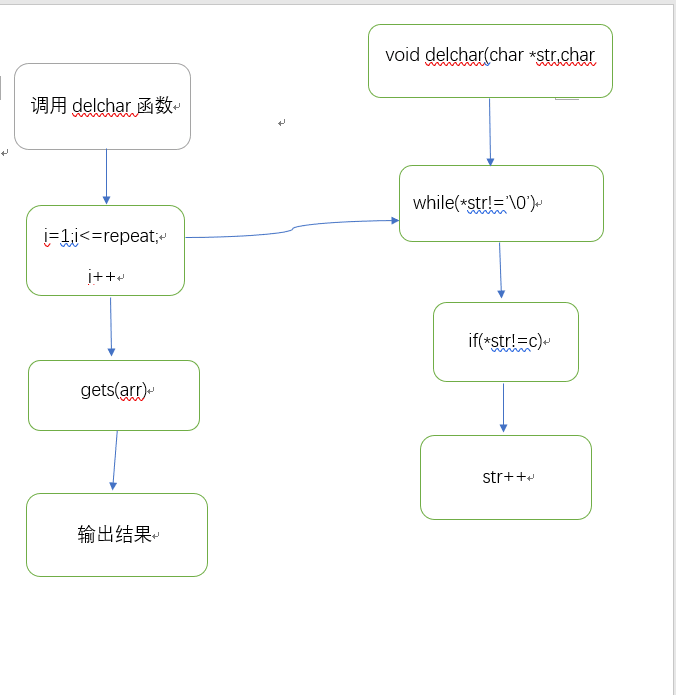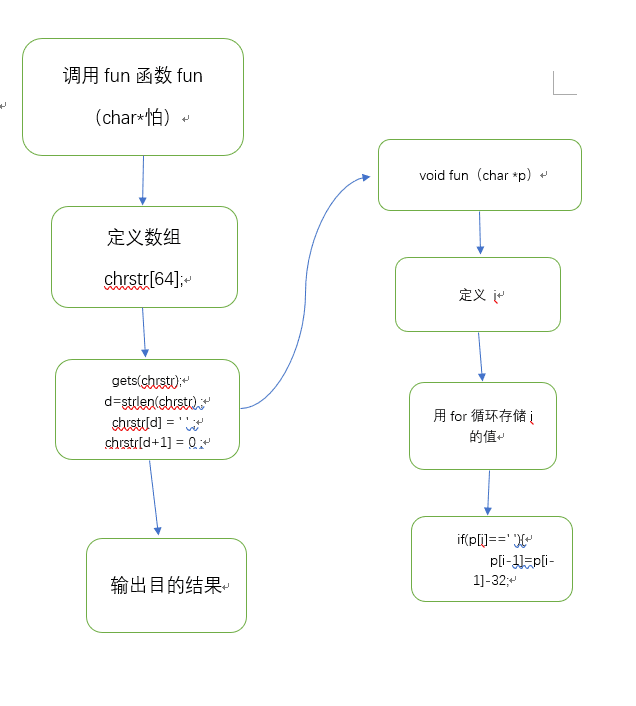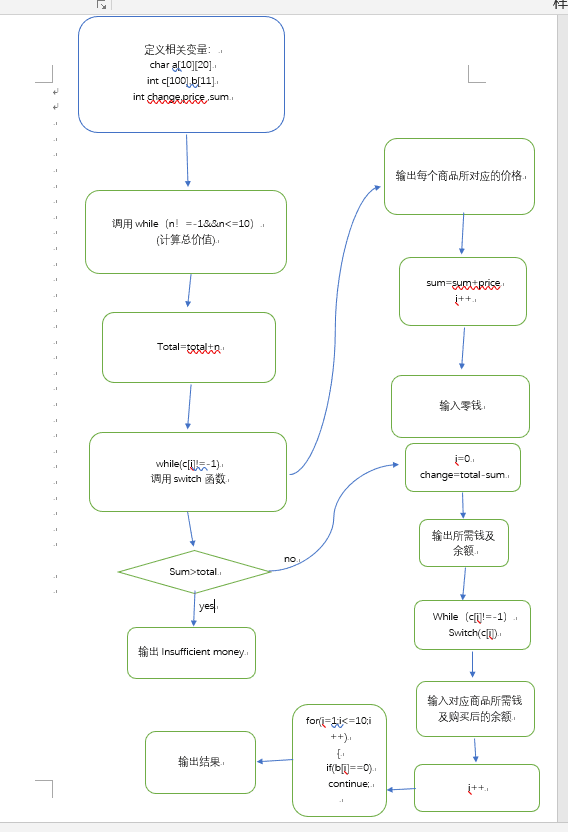# 2019春第7周作业

2019春第7周作业

 这个作业属于哪个课程 C语言程序设计Ⅱ 这个作业要求在哪里 https://edu.cnblogs.com/campus/zswxy/MS/homework/2829 我在这个课程的目标是 使用函数删除字符串中的字符 、利用指针及函数知识解决生活实际问题 在具体哪方面帮我实现目标 在处理类似自动售货机等有关问题 参考文献 C语言基础、https://www.bilibili.com/video/av384754?from=search&seid=14542647527198889976

7-1 使用函数删除字符串中的字符 （10 分)

``````3 (repeat=3）
happy new year (字符串"happy new year")
a (待删除的字符'a')
bee (字符串"bee")
e (待删除的字符'e')
111211 (字符串"111211")
1 (待删除的字符'1')``````

``````result: hppy new yer (字符串"happy new year"中的字符'a'都被删除)
result: b (字符串"bee"中的字符'e'都被删除)
result: 2 (字符串"111211"中的字符'1'都被删除)``````

``````#include<stdio.h>
void delchar(char *str,char c);
int main()
{
int repeat,i;
char arr,c;
scanf("%d\n",&repeat);
for(i=1;i<=repeat;i++)
{ gets(arr); scanf("%c",&c); printf("result: "); delchar(arr,c);
}
return 0;
} void delchar(char *str,char c)
{
while(*str!='\0')
{ if(*str!=c) putchar(*str); str++;
}
}``````6-2 每个单词的最后一个字母改成大写 （10 分)

``void fun( char *p );``

``````#include <stdio.h>
void fun( char *p );
int main()
{ char chrstr; int d ; gets(chrstr); d=strlen(chrstr) ; chrstr[d] = ' ' ; chrstr[d+1] = 0 ; fun(chrstr); printf("\nAfter changing: %s\n", chrstr);
return 0;
}``````

/* 请在这里填写答案 */

``my friend is happy``

``After changing: mY frienD iS happY``

``````#include <stdio.h>
void fun( char *p );
int main()
{ char chrstr; int d ; gets(chrstr); d=strlen(chrstr) ; chrstr[d] = ' ' ; chrstr[d+1] = 0 ; fun(chrstr); printf("\nAfter changing: %s\n", chrstr);
return 0;
}
void fun(char *p)
{ int i; for(i=0;p[i]!=0;i++){ if(p[i]==' '){ p[i-1]=p[i-1]-32; } }
}``````7-2 自动售货机 （30 分)

``````（1）从“钱币入口”放入钱币，依次放入多个硬币或纸币。钱币可支持1元（纸币、硬币）、2元（纸币）、5元（纸币）、10元（纸币），放入钱币时，控制器会先对钱币进行检验识别出币值，并统计币值总额，显示在控制器显示屏中，提示用户确认钱币放入完毕；
（2）用户确认钱币放入完毕，便可选择商品，只要用手指按对应商品外面的编号按钮即可。每选中一样商品，售货机控制器会判断钱币是否足够购买，如果钱币足够，自动根据编号将物品进行计数和计算所需钱币值，并提示余额。如果钱币不足，控制器则提示“Insufficient money”。用户可以取消购买，将会把所有放入钱币退回给用户。``````

``先输入钱币值序列，以-1作为结束，再依次输入多个购买商品编号，以-1结束。``

``输出钱币总额与找回零钱，以及所购买商品名称及数量。``

``````1 1 2 2 5 5 10 10 -1
1 2 3 5 1 6 9 10 -1``````

``````Total:36yuan,change:19yuan
Table-water:2;Table-water:1;Table-water:1;Milk:1;Beer:1;Oolong-Tea:1;Green-Tea:1;``````

``````#include<stdio.h>
int main(void)
{
int n,Total=0,i=0;
char a = {"Table-water","Table-water","Table-water","Coca-Cola","Milk","Beer","Orange-Juice","Sprite","Oolong-Tea","Green-Tea"};
int c,b={0,0,0,0,0,0,0,0,0,0,0};
int change,price,sum=0;
scanf("%d",&n);
while(n!=-1&&n<=10)
{ Total=Total+n; scanf("%d",&n);
}
scanf("%d",&c[i]);
while(c[i]!=-1)
{ switch(c[i]) { case 1:price=1;break; case 2:price=1;break; case 3:price=1;break; case 4:price=2;break; case 5:price=2;break; case 6:price=3;break; case 7:price=3;break; case 8:price=3;break; case 9:price=4;break; case 10:price=4;break; default:price=0;break; } sum=sum+price; i++; scanf("%d",&c[i]);
}
if(sum>Total)
printf("Insufficient money");
else
{ i=0; change=Total-sum; printf("Total:%dyuan,change:%dyuan\n",Total,change); while(c[i]!=-1) { switch(c[i]) { case 1: b++;break; case 2: b++;break; case 3: b++;break; case 4: b++;break; case 5: b++;break; case 6: b++;break; case 7: b++;break; case 8: b++;break; case 9: b++;break; case 10: b++;break; default:price=0;break; } i++; }
}
for(i=1;i<=10;i++)
{ if(b[i]==0) continue; printf("%s:%d;",a[i-1],b[i]);
} return 0;
} ``````日期 总代码行数 博客字数（个） 所花时间 知识点 03/03 39 781 4hour 查找整数（函数章节） 03/09 41 680 5hour 数组，文件 03/18 45 580 3hour 数组、指针 03/22 26+42+24=92 720 6hour 数组的综合运用 03/29 35+22=57 790 7hour 数组、指针 04/05 17+38+52=107 650 6hour 指针、数组、函数等 04/12 22+28+68=118 680 7hour 指针、数组、函数、分支结构等